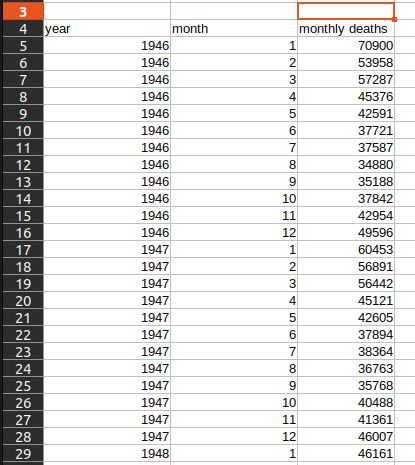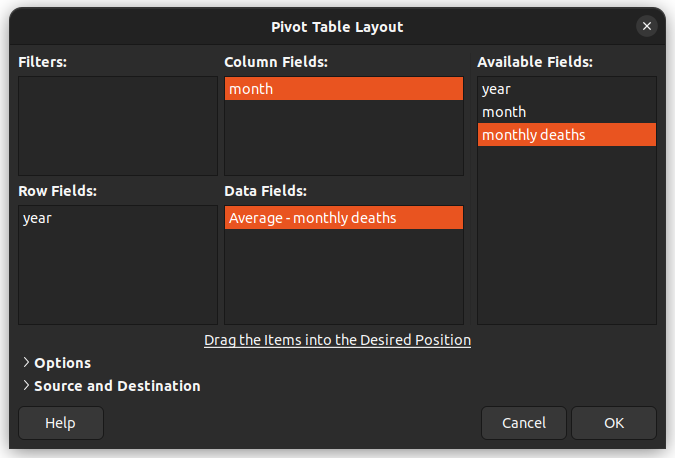# Convert monthly data to yearly data

Hi. I have monthly data that I would like to convert into yearly data, so I can plot a smoother graph with less points.

So the formula is pretty simple, but I tried filling it for 3 years and then dragging the formula, but it doesn"t work correctly.
The formula I typed in F7 is `=sum(B29:B40)`, and I want the formula in the next cell F8 to be `=sum(B41, B52)`, but when dragging the formula it puts `=SUM(B8:B19)` (so it takes the first fomula in F5 `=SUM(B5:B16)` and adds one for each column, instead of adding 12)

Is there an automated way to achieve what I want?
Alternatively, is there a way to plot ever 12th data point?

Have you considered using a pivot table, summing the monthly totals grouped by year, to use as the data source for your chart?

3 Likes

That could work, but if I understand pivot tables correctly, I would need to add a first column with a year every 12th line. And I’m having the same issue: prefilling a few values then dragging the selection does not automatically add the year as I expect.

2 Likes

Pivot table here is the best answer.
But if do you want a single value for year, you could enter in D5: `=SUM(B5:B16)`, select and copy D5:D16 (note that it is D, not B), and paste downward. This way you will get a yearly sum next to each January.

Thanks. Meanwhile, I just added year and month columns with formulas (`year = INT((ROW(A5) - 5) / 12 + 1946`, `month = MOD(ROW(C5) - 5, 12) + 1`), and was able to create the pivot table from this.Or you could have converted column A to dates, each being the first day of the relevant month.

[OT] Unrelated, I note that deaths in my birth month were significantly higher than any other month in the data you show. Yes, I am ancient